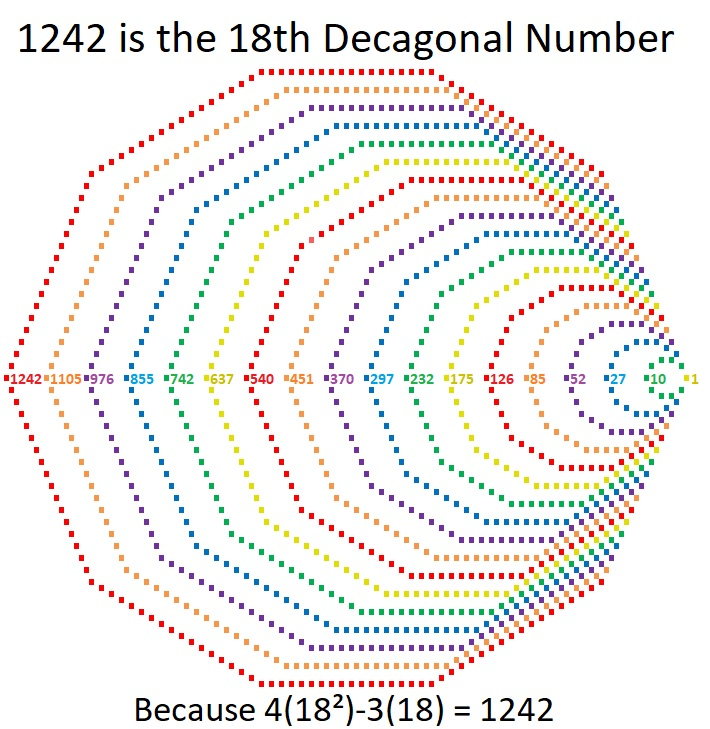# 1242 is a Decagonal Number

If you had 1242 tiny little squares you could arrange them into a decagon, just as I did for the graphic below.18 is a factor of 1242. Since 18(4·18-3) = 18(69) = 1242, it is the 18th decagonal number.

Here are a few more facts about the number 1242:

• 1242 is a composite number.
• Prime factorization: 1242 = 2 × 3 × 3 × 3 × 23, which can be written 1242 = 2 × 3³ × 23
• The exponents in the prime factorization are 1, 3, and 1. Adding one to each and multiplying we get (1 + 1)(3 + 1)(1 + 1) = 2 × 2 × 2 = 16. Therefore 1242 has exactly 16 factors.
• Factors of 1242: 1, 2, 3, 6, 9, 18, 23, 27, 46, 54, 69, 138, 207, 414, 621, 1242
• Factor pairs: 1242 = 1 × 1242, 2 × 621, 3 × 414, 6 × 207, 9 × 138, 18 × 69, 23 × 54, or 27 × 46
• Taking the factor pair with the largest square number factor, we get √1242 = (√9)(√138) = 3√138 ≈ 35.242021242 is the sum of consecutive prime numbers two different ways:
It is the sum of the eighteen prime numbers from 31 to 107, and
it is also the sum of the sixteen prime numbers from 43 to 109.